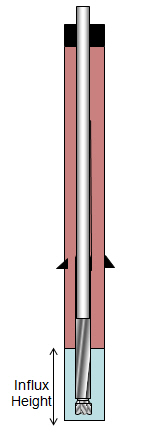# Calculate Influx Height

Once you know how much pit gain you got, you are able to determine influx height. Please take a look at the figure below for more understanding.The influx height can be calculated by this following equation.

## Influx height = Pit gain ÷ annular capacity

Where: influx height in ft,

Pit gain in bbl

Annular capacity in bbl/ft

The annular capacity can change depending on how big of your pipe and your hole.

Note: if you would like to get more understanding regarding annular capacity, please check this link, Annular Capacity Calculation.

Let’s get more understanding about it via this example.

Pit gain = 25 bbl

Hole size = 8.5 inch

BHA OD = 6 inch

BHA length = 420 ft

Drill pipe size = 5 inch

Firstly, you need to know annular capacity between BHA and hole.

Annular capacity, bbl/ft = (8.52 – 62) ÷ 1029.4 = 0.0325 bbl/ft

You can calculate how much annular volume between hole and drill collar via this following equation.

Annular Volume, bbl = BHA length x annular capacity

Annular Volume, bbl = 420 x 0.0325 = 14.8 bbl

Because you gain a total of 25 bbl, there will be some influx above the BHA.

Influx above the BHA, bbl = Total pit gain – Annular volume between hole and drill collar

Influx above the BHA, bbl = 25 – 14.8 = 10.2 bbl

Secondly, after knowing that 10.2 bbl of influx above the BHA, you need to convert this volume into height so as to determine height of the influx.

Determine annular capacity between drill pipe an hole.

Annular capaแity, bbl/ft = (8.52 – 52) ÷ 1029.4 = 0.0459 bbl/ft

Determine height of 10.2 bbl influx across 5” DP.

Height, ft = Volume, bbl ÷ Annular Capacity, bbl/ft

Height, ft = 10.2 ÷ 0.0459 = 222 ft

It means that there is 222 ft of influx across DP so you can determine the total influx height:

Height of influx = 222 + 420 = 642 ft

References

Cormack, D. (2007). An introduction to well control calculations for drilling operations. 1st ed. Texas: Springer.

Crumpton, H. (2010). Well Control for Completions and Interventions. 1st ed. Texas: Gulf Publishing.

Share the joy